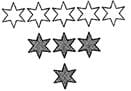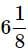Test : Fractions - 2

# Test : Fractions - 2

Test Description

## 10 Questions MCQ Test Mathematics for Class 5: NCERT | Test : Fractions - 2

Test : Fractions - 2 for Class 5 2023 is part of Mathematics for Class 5: NCERT preparation. The Test : Fractions - 2 questions and answers have been prepared according to the Class 5 exam syllabus.The Test : Fractions - 2 MCQs are made for Class 5 2023 Exam. Find important definitions, questions, notes, meanings, examples, exercises, MCQs and online tests for Test : Fractions - 2 below.
Solutions of Test : Fractions - 2 questions in English are available as part of our Mathematics for Class 5: NCERT for Class 5 & Test : Fractions - 2 solutions in Hindi for Mathematics for Class 5: NCERT course. Download more important topics, notes, lectures and mock test series for Class 5 Exam by signing up for free. Attempt Test : Fractions - 2 | 10 questions in 10 minutes | Mock test for Class 5 preparation | Free important questions MCQ to study Mathematics for Class 5: NCERT for Class 5 Exam | Download free PDF with solutions
 1 Crore+ students have signed up on EduRev. Have you?
Test : Fractions - 2 - Question 1

### Represent the shaded stars out of total stars in the following figure as a fraction.Test : Fractions - 2 - Question 2

### In which one of the following figures shaded part represents 1/16?

Test : Fractions - 2 - Question 3

### Two fractions are called like fractions, if they have same:

Test : Fractions - 2 - Question 4

a/b is a fraction. If a is smaller than b, a/b is a:

Test : Fractions - 2 - Question 5

Convert the fraction 209/12 into a mixed faction.

Test : Fractions - 2 - Question 6

Convert the mixed fractioninto an improper fraction.

Test : Fractions - 2 - Question 7

Represent the shaded part in the figure given in the options as a fraction and choose the correct option.

Test : Fractions - 2 - Question 8

Which one of the following options has a pair of equivalent fraction?

Test : Fractions - 2 - Question 9

Write the fraction 90/145 in its lowest form.

Test : Fractions - 2 - Question 10

Which one of the following is an equivalent fraction of 12/27 ?

## Mathematics for Class 5: NCERT

36 videos|73 docs|45 tests
 Use Code STAYHOME200 and get INR 200 additional OFF Use Coupon Code
Information about Test : Fractions - 2 Page
In this test you can find the Exam questions for Test : Fractions - 2 solved & explained in the simplest way possible. Besides giving Questions and answers for Test : Fractions - 2, EduRev gives you an ample number of Online tests for practice

## Mathematics for Class 5: NCERT

36 videos|73 docs|45 tests